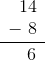# ISEE Lower Level Quantitative : How to subtract

## Example Questions

### Example Question #367 : Isee Lower Level (Grades 5 6) Quantitative Reasoning

The swing set isinches tall and the tree is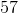inches tall. How much taller is the tree than the swing set?inchesinches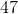inchesinchesinchesinches

Explanation:

This is a subtraction problem because we want to know how much taller the tree is compared to the swing set, or the difference in their height.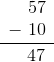### Example Question #368 : Isee Lower Level (Grades 5 6) Quantitative Reasoning

The remote isinches long and the TV isinches long. How much longer is the TV than the remote?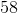inches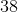inchesinchesinchesinchesinches

Explanation:

This is a subtraction problem because we want to know how much longer the TV is compared to the remote, or the difference in their length.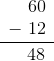### Example Question #369 : Isee Lower Level (Grades 5 6) Quantitative Reasoning

The dog’s body isinches long and his tail isinches long. How much longer is his body than his tail?inchesinchesinches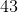inchesinchesinches

Explanation:

This is a subtraction problem because we want to know how much longer the dog's body is compared to his tail, or the difference in their length.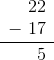### Example Question #370 : Isee Lower Level (Grades 5 6) Quantitative Reasoning

The coffee cup isinches tall and the water glass isinches tall. How much taller is the water glass than the coffee cup?inchesinchesinchesinchesinchesinches

Explanation:

This is a subtraction problem because we want to know how much taller the water glass is compared to the coffee cup, or the difference in their height.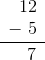### Example Question #55 : Measurement & Data

The cat isinches long and the dog isinches long. How much longer is the dog than the cat?inchesinchesinchesinchesinchesinches

Explanation:

This is a subtraction problem because we want to know how much longer the dog is compared to the cat, or the difference in their length.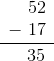### Example Question #56 : Measurement & Data

The bowl isinches long and the plate isinches long. How much longer is the plate than the bowl?inchesinchesinchesinchesinchesinches

Explanation:

This is a subtraction problem because we want to know how much longer the plate is compared to the bowl, or the difference in their length.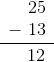### Example Question #11 : Use Addition And Subtraction Within 100 To Solve Word Problems Involving Lengths: Ccss.Math.Content.2.Md.B.5

The swing set isinches tall and the tree is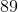inches tall. How much taller is the tree than the swing set?inchesinches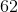inchesinches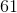inchesinches

Explanation:

This is a subtraction problem because we want to know how much taller the tree is compared to the swing set, or the difference in their height.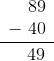### Example Question #58 : Measurement & Data

The remote isinches long and the TV isinches long. How much longer is the TV than the remote?inchesinchesinchesinchesinchesinches

Explanation:

This is a subtraction problem because we want to know how much longer the TV is compared to the remote, or the difference in their length.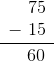### Example Question #59 : Measurement & Data

The dog’s body isinches long and his tail isinches long. How much longer is his body than his tail?inchesinchesinchesinchesinchesinches

Explanation:

This is a subtraction problem because we want to know how much longer the dog's body is compared to his tail, or the difference in their length.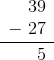### Example Question #60 : Measurement & Data

The coffee cup isinches tall and the water glass isinches tall. How much taller is the water glass than the coffee cup?inchesinchesinchesinhcesinchesinches